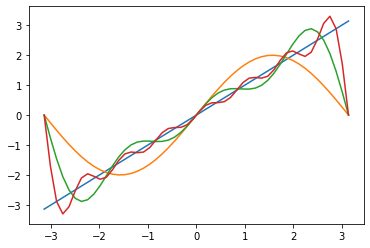# Python (SymPy) Programming for Fourier analysis

This article deals with the Fourier expansions using SymPy, a Python library for symbolic calculations. An example in this article provides an identity for pi, which will be examined numerically by Numpy, a Python library for numerical calculations. An introductory collection of SymPy usage can be found in the previous article:

#### Orthogonality of trigonometric functions

The following set of functionsprovides an orthonormal basis for functions defined on a region. One can check it by running the following code on Python:

import sympy as sp

x = sp.symbols('x')
def c(m, x):
if m == 0: return 1/sp.sqrt(2*sp.pi)
else: return sp.cos(m*x)/sp.sqrt(sp.pi)
def s(m, x): return sp.sin(m*x)/sp.sqrt(sp.pi)

def cc(m,n): return sp.integrate(c(m,x)*c(n,x), (x, -sp.pi, sp.pi))
def cs(m,n): return sp.integrate(c(m,x)*s(n,x), (x, -sp.pi, sp.pi))
def ss(m,n): return sp.integrate(s(m,x)*s(n,x), (x, -sp.pi, sp.pi))


Then, for example

CC = [[],[],[],[],[]]
for m in range(5):
for n in range(5):
CC[m].append(cc(m,n))
print(CC)


shows (for)SIimilarly

SS = [[],[],[],[],[]]
for m in range(5):
for n in range(5):
SS[m].append(ss(m,n))
print(SS)


shows (for)and finally

CS = [[],[],[],[],[]]
for m in range(5):
for n in range(5):
CS[m].append(cs(m,n))
print(CS)


showsThus one may be able to convince yourself that the orthonormality of the set of functions.

#### Fourier series expansions

A series expansion of a functiondefined on a regiongiven in the following formis called Fourier expansion of. Owing to the orthonormality of the functions, the coefficients are determined by#### Example

##### f(x)=xsp.init_printing()
def f(x): return x
def a(n): return sp.integrate(c(n,x)*f(x), (x, -sp.pi, sp.pi))
def b(n): return sp.integrate(s(n,x)*f(x), (x, -sp.pi, sp.pi))
A = []
B = []
for n in range(10):
A.append(a(n))
B.append(b(n))
[A,B]


givesthus its Fourier expansion is given by##### Built-in: fourier_series

While SymPy has a built-in command for obtaining Fourier coefficients:

ff = sp.fourier_series(f(x), (x, -sp.pi, sp.pi)).truncate(8)
# truncate( ) specifies how many order to expand
ff


##### Drawing a graph

To visualize how much extent the expansion approximates the function, let it draw the graphs of expansion truncated:

import matplotlib.pyplot as plt
import numpy as np

D = 50
xmin = -np.pi
xmax = np.pi

def Ff(n, x):
return sp.fourier_series(f(x), (x, -sp.pi, sp.pi)).truncate(n)
p = np.linspace( xmin, xmax, D)
def FF(n,k): return float(Ff(n, x).subs(x, p[k]))
Q1 = []
Q3 = []
Q7 = []
for k in range(D):
Q1.append(FF(1,k))
Q3.append(FF(3,k))
Q7.append(FF(7,k))
q = p
q1 = np.array(Q1)
q3 = np.array(Q3)
q7 = np.array(Q7)

plt.plot(p ,q)
plt.plot(p ,q1)
plt.plot(p ,q3)
plt.plot(p ,q7)
plt.show()


provideswhere blue line is for the original function, and orange, green and red, are accordingly truncations at order, including higher modes.

##### Pi (Leibniz/Euler's series)

The Fourier expansion obtained above implies, by setting, the following identity:This series is referred to as Leibniz's series or Euler's series. Examining this series numerically shall be done by running the following code:

k = sp.var('k', integer = True)
def p(n): return 4+4*sp.summation((-1)**k/(2*k+1), (k, 1, n))


gives a finite sum of the series till-th term. For example, 1,000 terms gives

float(p(1000))while the actual value is

float(sp.pi)Then one finds that 1,000＝terms reproduce it 3-digit accuracy.

10,000 terms

float(p(10000))improves it 1-digit, but it takes computer resources and takes some time to evaluate.

Using a list gives a faster computation:

N = 10000
P = 
def q(k): return 4*(-1)**k/(2*k+1) + P[k-1]
for k in range(1,N):
P.append(q(k))
P[N-1]


forN = 10**7
P = 
def q(k): return 4*(-1)**k/(2*k+1) + P[k-1]
for k in range(1,N):
P.append(q(k))
P[N-1]


givesso that the accuracy seems improved by inclusions of more terms.

Keywords: Python, SymPy, Fourier series, Orthonormal functions